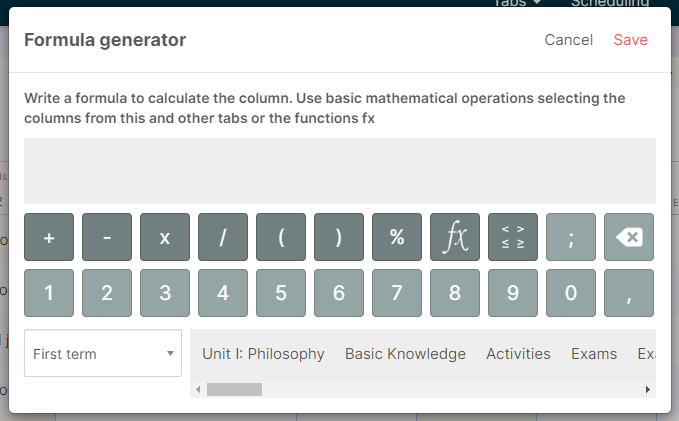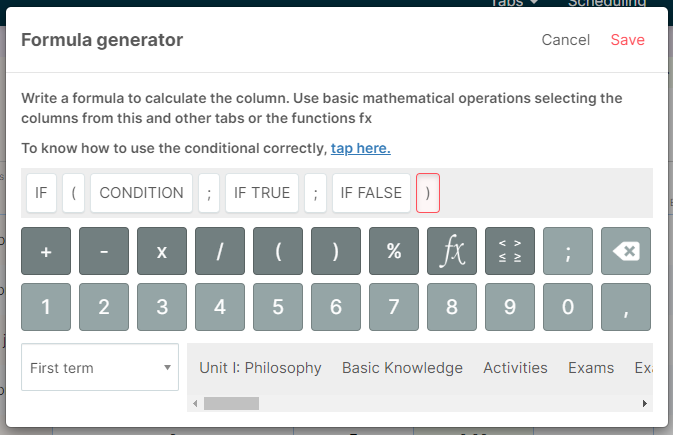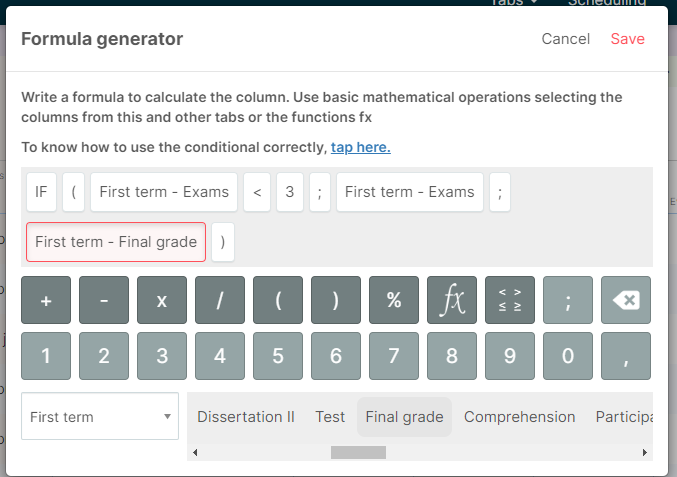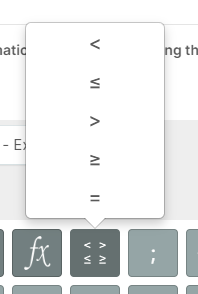1. Help center - Additio App

# Conditionals: Still more possibilities of calculations in Additio

Sometimes, the result of an exam can determine if the student deserves to calculate the final grade along with the rest of activities or he doesn't. In Additio, you can do this calculation with conditionals.

You can use conditionals in Calculated formulas, once the option Calculated formula has been selected on type of evaluation > Calculations. By defining this option, you'll be able to establish a range of grades that can affect the final result.

To create this type of calculation, you have to create a column with Calculated formula option and select fx icon to set the conditional.Select Conditional and a condition will be displayed in the field. You can adapt it according to a grade condition.To set this correctly, here we have an example:

In my classes, if the result of an exam is not over 3, there is no average to consider with the exercises done during the course, so the grade of the exam becomes the final grade.

We need to consider the following sections: CONDITION (the conditional we want to apply), IF TRUE (what happens if the result of the exam is under 3), IF FALSE (what happens if the result of the exam is over 3).